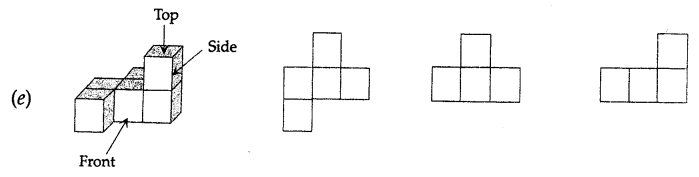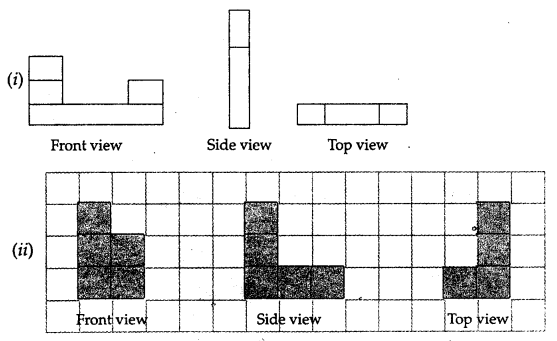## ML Aggarwal Class 7 Solutions for ICSE Maths Chapter 15 Visualising Solid Shapes Ex 15.3

Question 1.
Count the number of cubes in the following structures:Solution:
Number of cubes in the given structure havebeen given below:
(i) 5 × 3 × 2 = 30
(ii) 7 × 2 + 6 = 14 + 6 = 20
(iii) 5 × 5 + 17 + 4 = 25 + 17 + 4 = 46

Question 2.
What cross-section is made in the following
(i) vertical cut (ii) horizontal cut?
(a) A brick
(b) A round apple
(c) A die
(d) A circular pipe
(e) An ice cream cone
(f) A square pyramid
Solution:

 Vertical cut Horizontal cut (a) A brick Rectangle Rectangle (b) A round apple Circle Circle (c) A die Square Square (d) A circular pipe Rectangle Circle (e) An ice cream cone Triangle Circle (f) A square pyramid Triangle Square

Question 3.
For each solid given below, the three views (1), (2) and (3) are given. Identify for each solid the corresponding top, front and side views:Solution:
(a) (1) Side (2) Top (3) Front
(b) (1) Front (Top) (2) Side (3) Top (Front)
(c) (1) Top (2) Side (3) Front
(d) (1) Front (Side) (2) Side (Front) (3) Top
(e) (1) Top (2) Side (3) Front

Question 4.
For the soldis given below sketch the front, side and top view:Solution: